# EfficientNet Trained onImageNet with AdvProp and AutoAugment

Identify the main object in an image

Released in 2019, this model utilizes the techniques of AdvProp and AutoAugment data augmentation on the EfficientNet architectures to effectively perform image classification.

Number of models: 8

## Performance

• The models achieve the following accuracies on the original ImageNet validation set.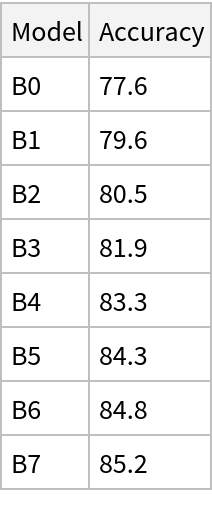## Examples

### Resource retrieval

Get the pre-trained net:

 In:=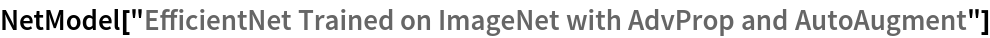Out=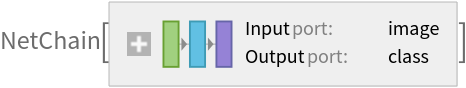### NetModel parameters

This model consists of a family of individual nets, each identified by a specific parameter combination. Inspect the available parameters:

 In:=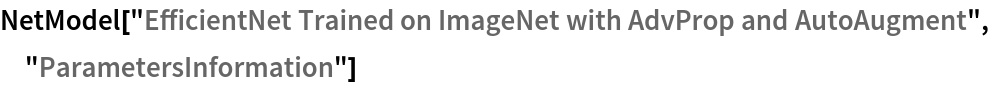Out=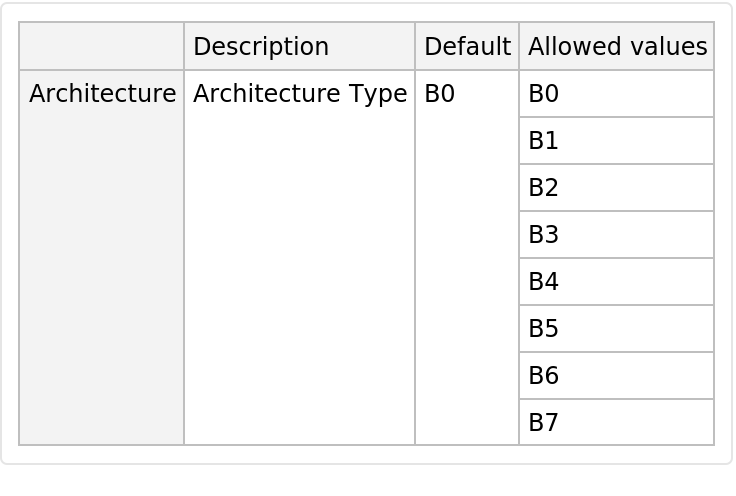Pick a non-default net by specifying the parameters:

 In:=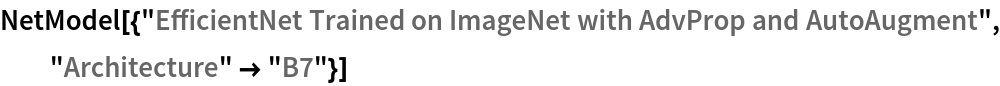Out=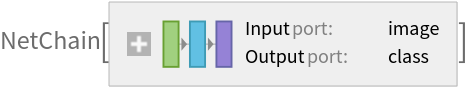Pick a non-default uninitialized net:

 In:=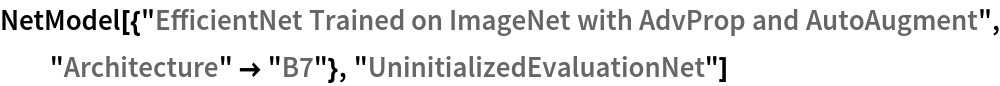Out=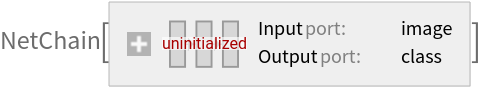### Basic usage

Classify an image:

 In:=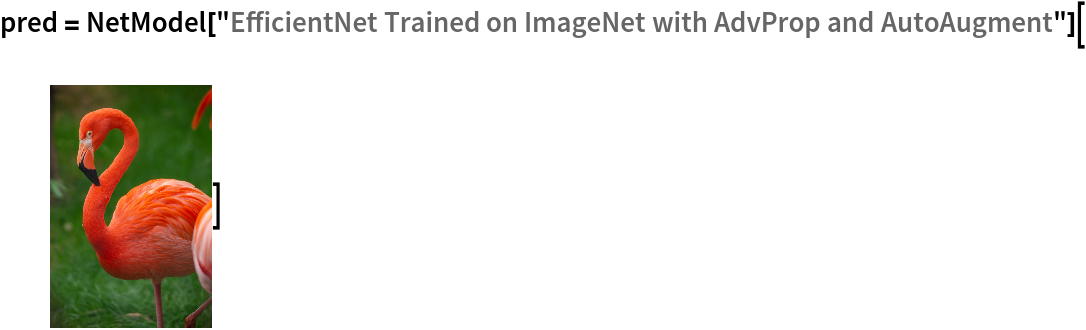Out=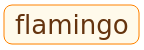The prediction is an Entity object, which can be queried:

 In:=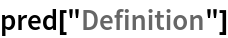Out=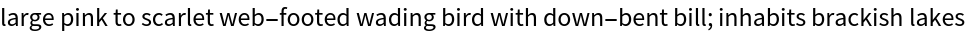Get a list of available properties of the predicted Entity:

 In:=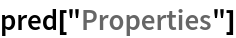Out=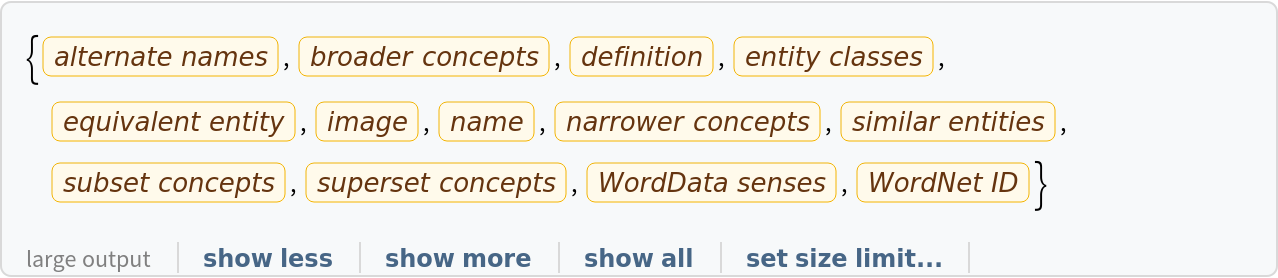Obtain the probabilities of the 10 most likely entities predicted by the net:

 In:=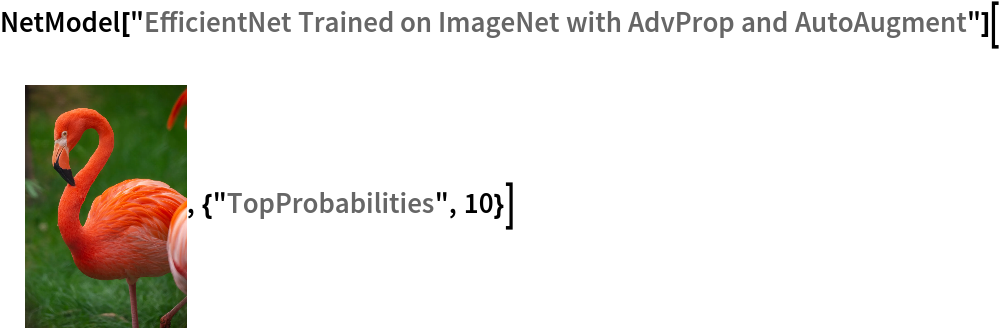Out=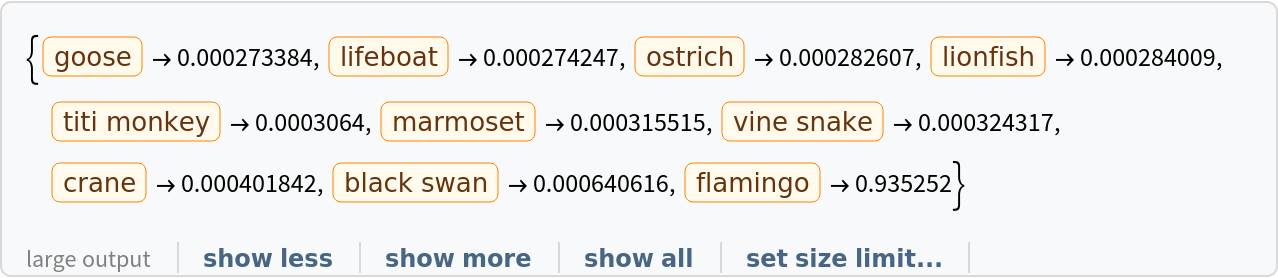An object outside the list of the ImageNet classes will be misidentified:

 In:=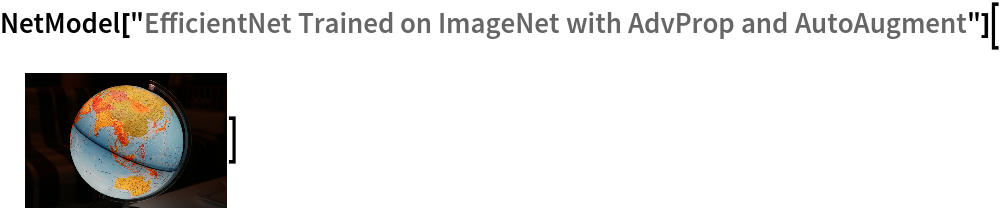Out=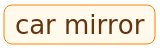Obtain the list of names of all available classes:

 In:=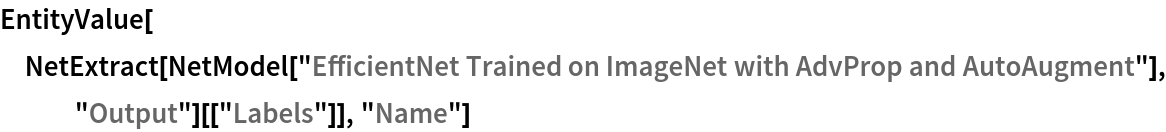Out=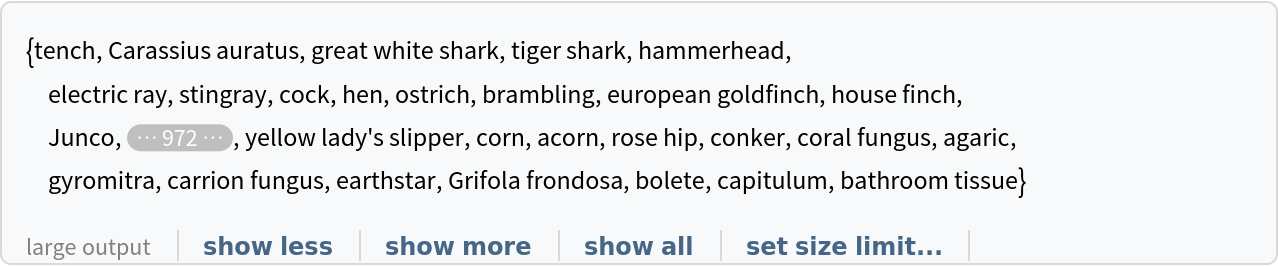### Feature extraction

Remove the last two layers of the trained net so that the net produces a vector representation of an image:

 In:=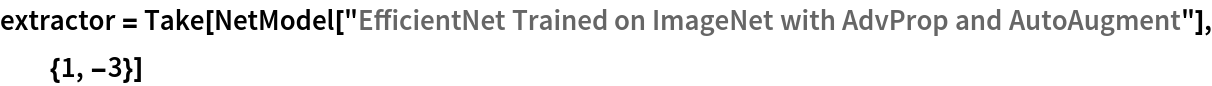Out=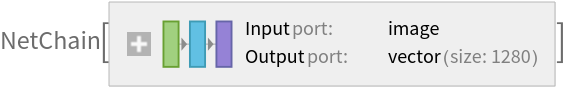Get a set of images:

 In:=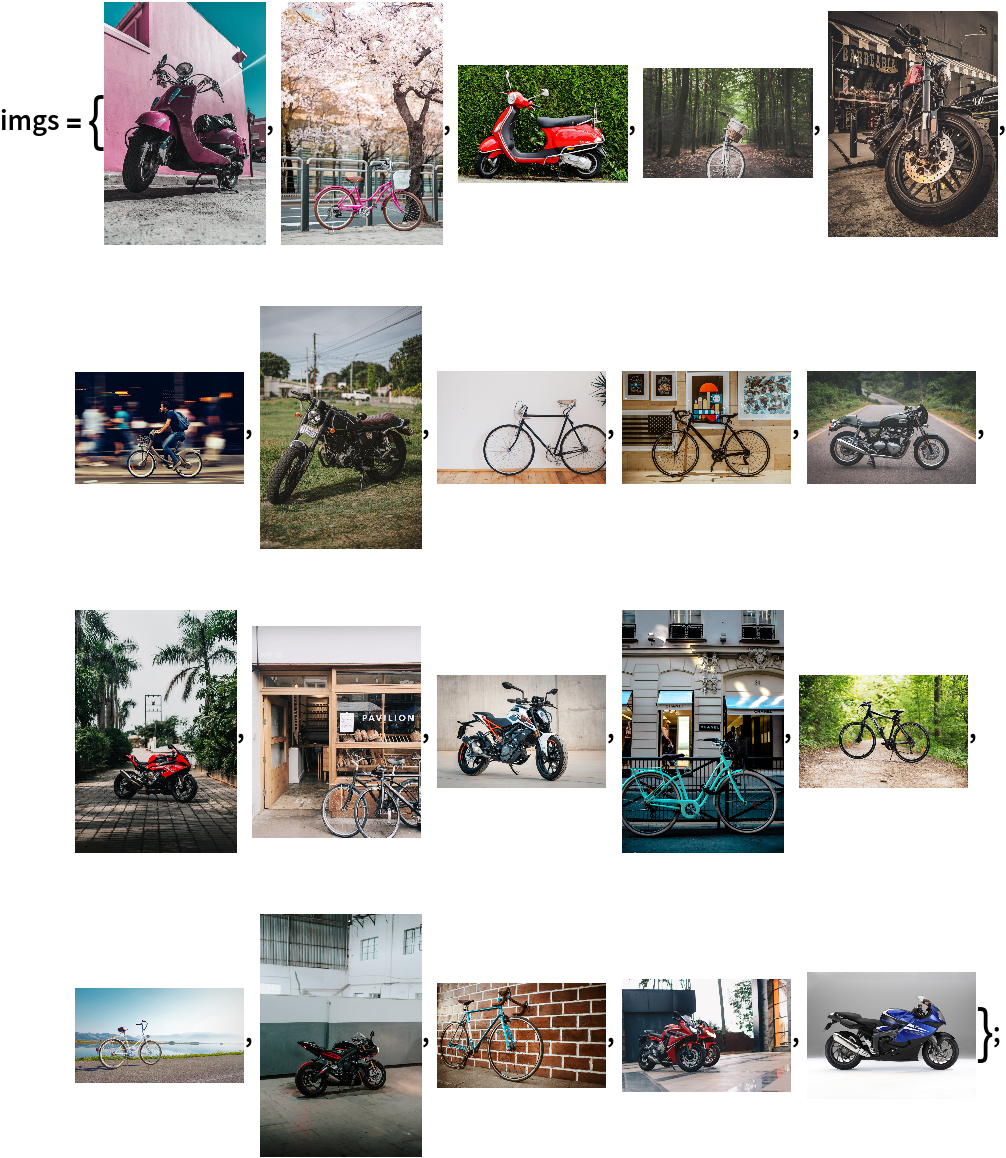Visualize the features of a set of images:

 In:=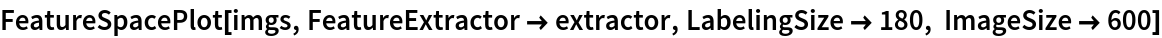Out=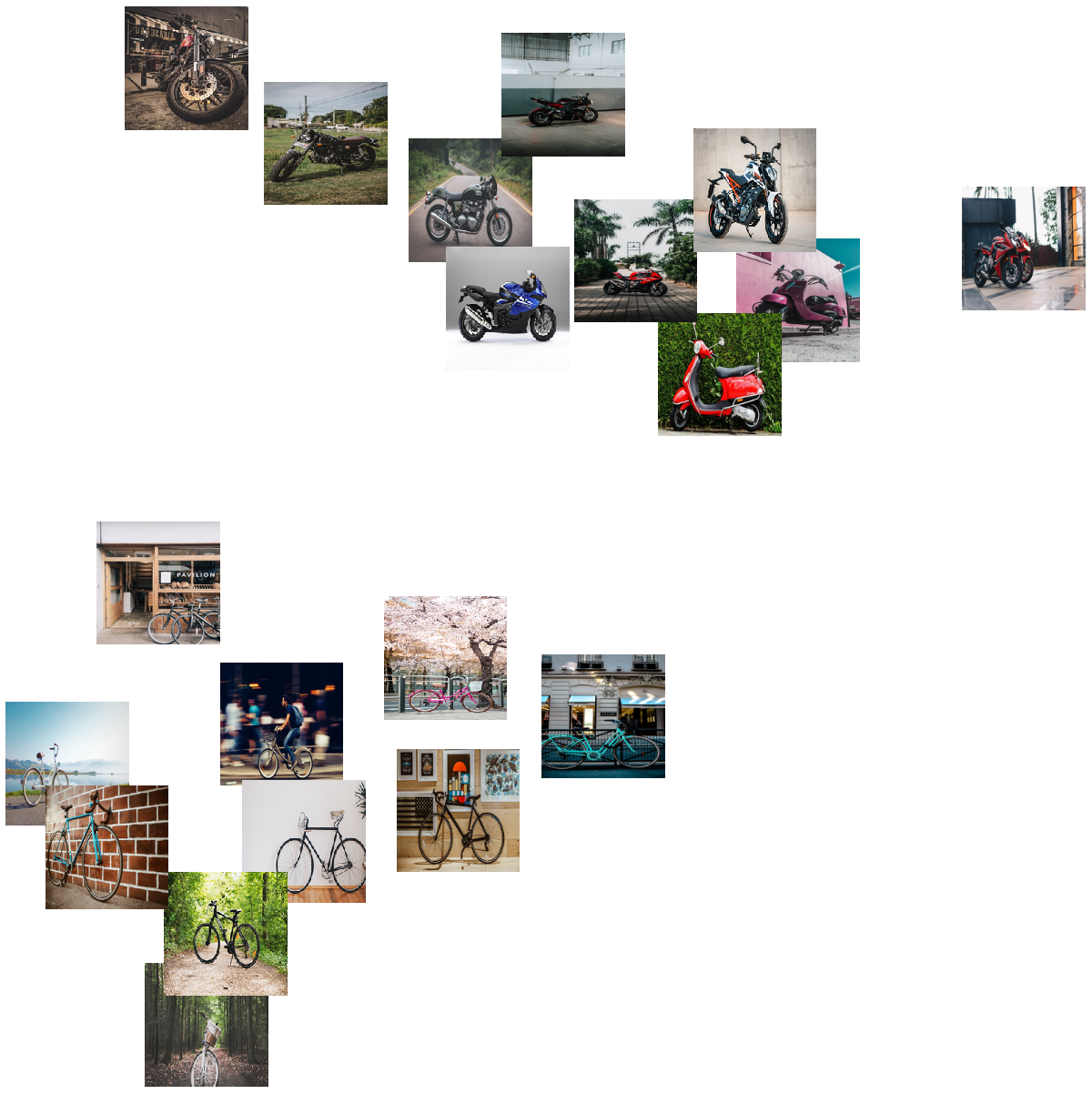### Visualize convolutional weights

Extract the weights of the first convolutional layer in the trained net:

 In:=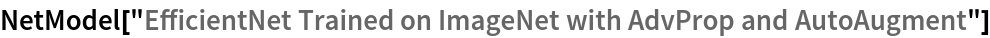Out=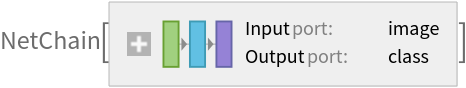In:=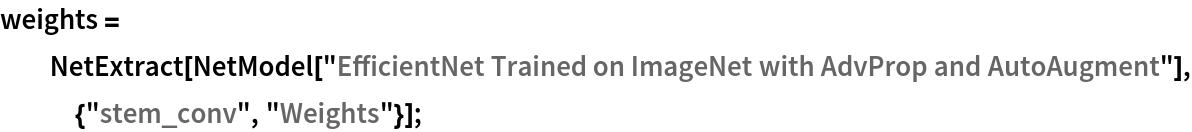Show the dimensions of the weights:

 In:=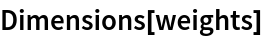Out=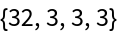Visualize the weights as a list of 32 images of size 3x3:

 In:=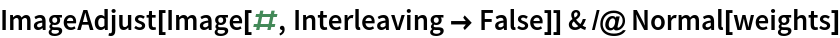Out=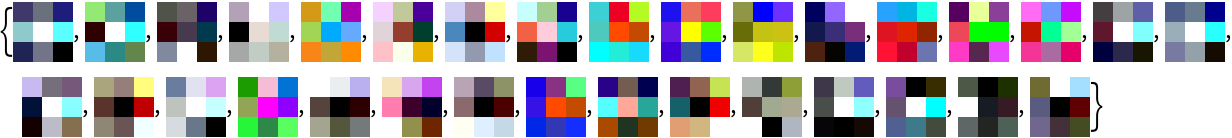### Transfer learning

Use the pre-trained model to build a classifier for telling apart images of motorcycles and bicycles. Create a test set and a training set:

 In:=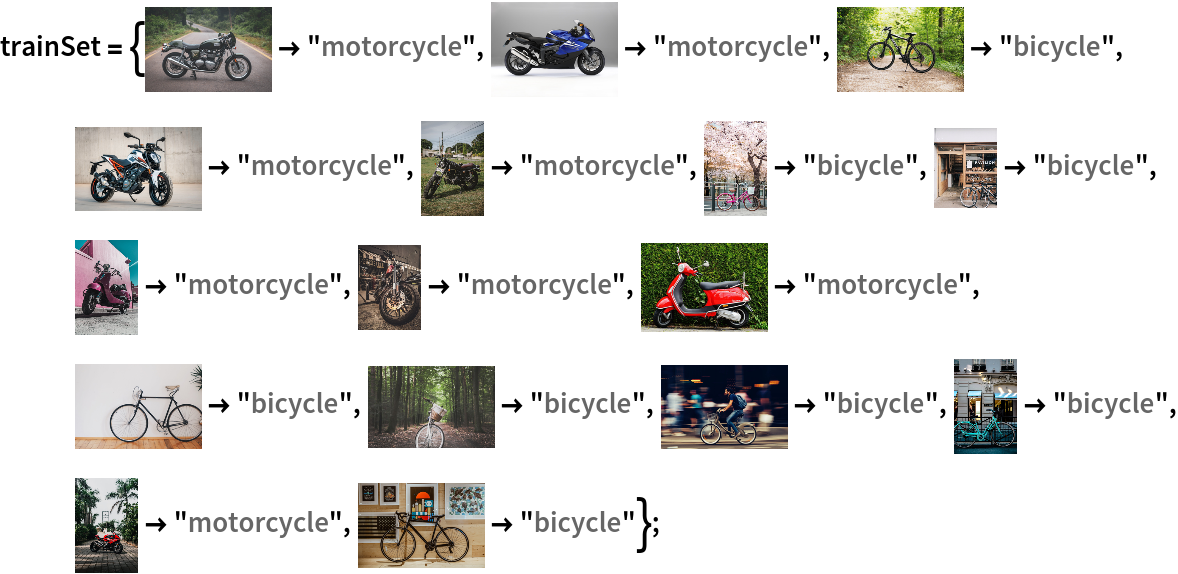In:=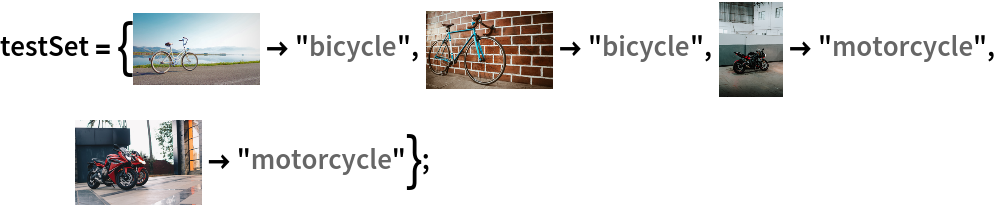Remove the linear layer from the pre-trained net:

 In:=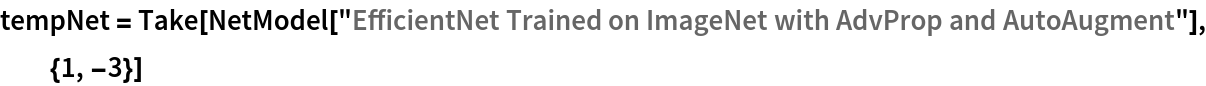Out=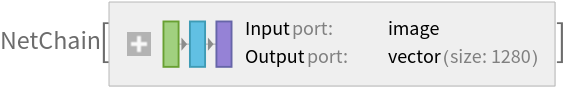Create a new net composed of the pre-trained net followed by a linear layer and a softmax layer:

 In:=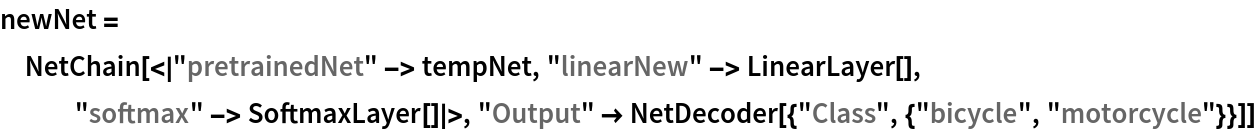Out=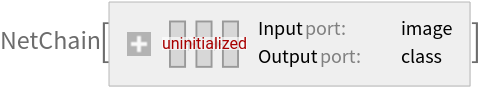Train on the dataset, freezing all the weights except for those in the "linearNew" layer (use TargetDevice -> "GPU" for training on a GPU):

 In:=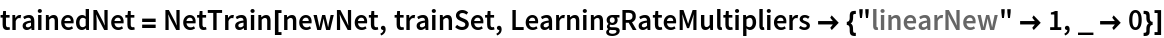Out=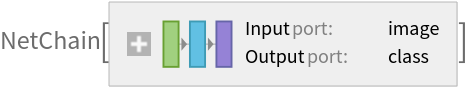Perfect accuracy is obtained on the test set:

 In:=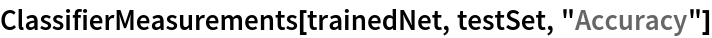Out=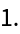### Net information

Inspect the number of parameters of all arrays in the net:

 In:=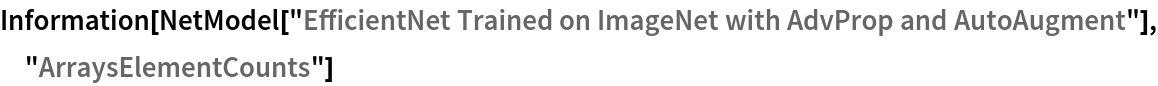Out=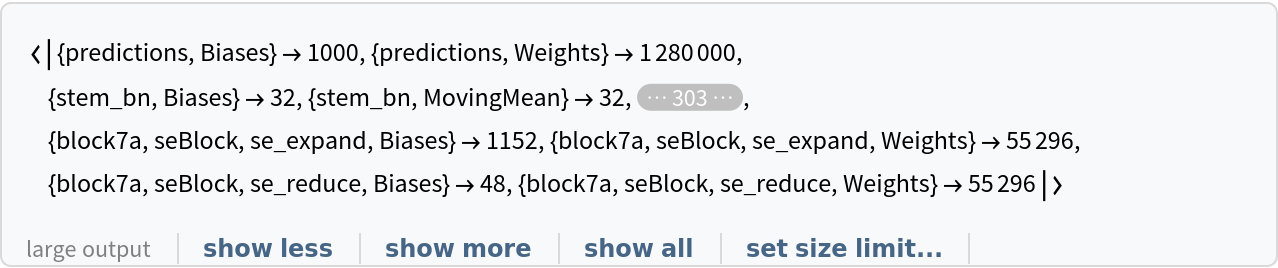Obtain the total number of parameters:

 In:=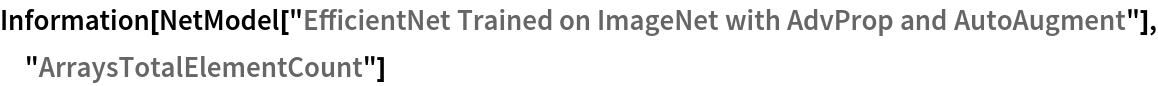Out=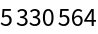Obtain the layer type counts:

 In:=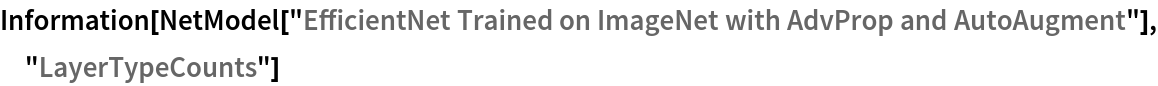Out=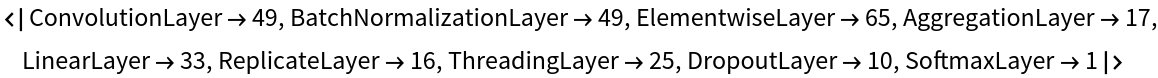### Export to MXNet

Export the net into a format that can be opened in MXNet:

 In:=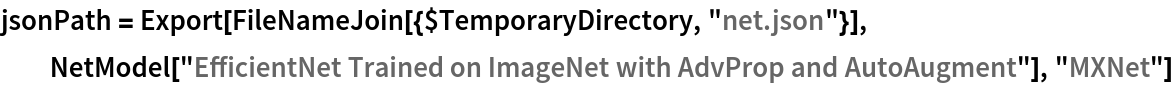Out=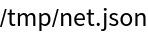Export also creates a net.params file containing parameters:

 In:=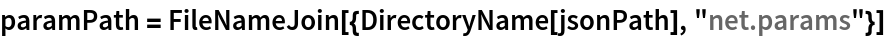Out=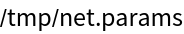Get the size of the parameter file:

 In:=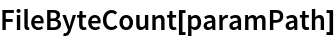Out=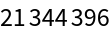## Requirements

Wolfram Language 12.1 (March 2020) or above

## Reference

• E. Cubuk, B. Zoph, D. Mane, V. Vasudevan, Q. Le, "AutoAugment: Learning Augmentation Policies from Data," arXiv:1805.09501 (2018)
M. Tan, Q. Le, "EfficientNet: Rethinking Model Scaling for Convolutional Neural Networks," arXiv:1905.11946 (2019)
C. Xie, M. Tan, B. Gong, J. Wang, A. Yuille, Q. Le, "Adversarial Examples Improve Image Recognition," arXiv:1911.09665 (2019)